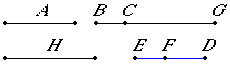# Proposition 86

To find the second apotome.

Set out a rational straight line A, and let GC be commensurable in length with A. Then GC is rational. Set out two square numbers DE and EF, and let their difference DF not be square.

X.6,Cor.

Now let it be contrived that FD is to DE as the square on CG is to the square on GB.X.6

Then the square on CG is commensurable with the square on GB.

X.9

But the square on CG is rational, therefore the square on GB is also rational. Therefore BG is rational. And, since the square on GC does not have to the square on GB the ratio which a square number has to a square number, therefore CG is incommensurable in length with GB.

X.73

And both are rational, therefore CG and GB are rational straight lines commensurable in square only. Therefore BC is an apotome.

I say next that it is also a second apotome.

Let the square on H be that by which the square on BG is greater than the square on GC.

V.19,Cor.

Since the square on BG is to the square on GC as the number ED is to the number DF, therefore, in conversion, the square on BG is to the square on H as DE is to EF.

X.9

And each of the numbers DE and EF is square, therefore the square on BG has to the square on H the ratio which a square number has to a square number. Therefore BG is commensurable in length with H.

And the square on BG is greater than the square on GC by the square on H, therefore the square on BG is greater than the square on GC by the square on a straight line commensurable in length with BG.

X.Def.III.2

And CG, the annex, is commensurable with the rational straight line A set out, therefore BC is a second apotome.

Therefore the second apotome BC has been found.

Q.E.F.

## Guide

This proposition is not used in the rest of the Elements.